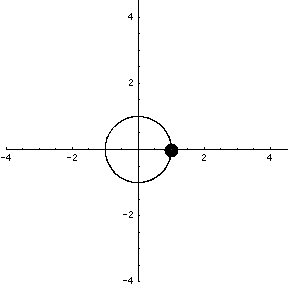# Math Insight

### Image: Parametrization of a circle 4An animation of the parametrization $\adllp(t) = (\cos(t^2), \sin(t^2))$ of the unit circle, with dot $\adllp(t)$ at time time and vector $\adllp'(t)$ showing velocity.

Image file: parametrization_circle_4.gif